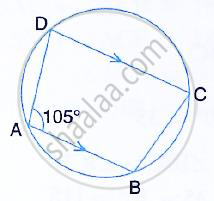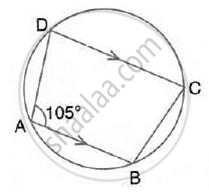Share

In the Figure, Given Below, Find: (I) ∠Bcd, (Ii) ∠Adc, (Iii) ∠Abc, Show Steps of Your Working . - ICSE Class 10 - Mathematics

Question

In the figure, given below, find: (i) ∠BCD, (ii) ∠ADC, (iii) ∠ABC, Show steps of your working .Solution(i) ∠BCD + ∠BAD = 180°
(Sum of opposite angles of a cyclic quadrilateral is 180° )
⇒ ∠BCD= 180° -105° = 75°

(ii) Now, AB ∥ CD